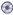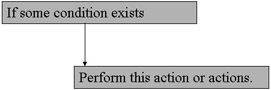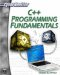# Chapter 5: Decision Structures and LoopsDownload CD Content

By now you should have a firm grasp of essentially what C++ is, and know how to create a variable, use functions, and write some simple C++ programs. Now it’s time to add to that knowledge, and expand your programming skill-set. This chapter will introduce you to decision structures and loops. These are key concepts that exist in all programming languages; it is just their implementation that is somewhat different. In this chapter, you will learn how to have your code branch in different directions based on certain criteria. You will also learn how to have your code loop repeatedly. A decision structure is a block of code that branches, depending on some decision. There are many times when you will need different code to execute, depending on some criteria such as user input. You have probably used programs in which you were asked to provide some input, maybe a simple yes or no. The program behaved differently based on the input you gave it. This is an example of a decision structure. Different blocks of code are executed differently based on some criteria. Essentially a decision structure is code that lets your code branch based on some criteria.

Most programs are simply combinations of decisions. If the user does this, then the program responds with one action. If the user does something else, then the program responds with a different action. The entire activity of data processing is dominated by decisions. For this reason, decision structures are a key programming concept found in all programming languages.

## If Statements

The most common type of decision structure is the if statement. These exist in all programming languages but are implemented slightly differently. An if statement literally says, “if some condition exists, then do this certain code.” A generic, programming-language-neutral example would be the following.

`If some condition exists  Execute this code`

The condition is usually the value of some variable. If a particular variable contains a certain value, or even a range of values, then execute a given block of code. The diagram shown in Figure 5.1A shows the basic structure and flow of an if statement.Figure 5.1A: The structure of an if statement.

It is important to recall that if statements are a common facet of almost all programming languages. In every case they will follow the structure and flow shown in Figure 5.1A, but the specific way a particular programming language might go about implementing this concept can be quite different from other languages. However, you should know that many languages use syntax that is similar to the syntax of C++. In fact, if statements in C, Java, and JavaScript are almost indistinguishable from if statements in C++. This is important for you to realize. The more thoroughly you master the C++ programming language, the easier it will be for you to learn these other programming languages at some future data, should you so desire.

Let’s look at how you implement if statements in C++. Here is a basic example that we can dissect to help you learn about if statements.

`If( age == 16) {   cout << "Yahoo... Your old enough to drive!\n"; }`

Let’s take a look at this code because there are several things you should note. First of all, you have the word if followed by parentheses. Inside the parentheses is where you locate the condition that you base your if-statement on. In other words, if (whatever is in the parentheses is true) do the stuff in the following brackets. The brackets, recall from our discussion of functions, are just boundaries around any block of code. The code in these brackets is what you wish to execute if the statement in the parentheses is true. Finally, notice that there is a double equals sign in the parentheses. Think back to Chapter 1 where you were first introduced to operators. Remember that a single equals is an assignment operator and a double equals is an evaluation operator. By using the double equals, you are asking “is the age equal to 16?” If you used the single equals, you would be stating, “make the age equal to 16.”

 Watchout! One of the most common mistakes that beginners make is to use a single equals (assignment operator) in an if statement rather than a double equals. If you use the single equals—the assignment operator instead of the double equals—the equality operator—you will make the if statement true every time.

### Example 5.1

Let’s use an if statement in a simple program. What we are going to do is to use a simple menu displayed on the screen, and then proceed based on what the user selects.

Step 1: Type the following code into your favorite text editor. Save it as05-01.cpp.

`#include <iostream> using namespace std; float square_num(float num); float cube_num(float num); int main() {   int choice;   float number, answer;   cout << "Would you like to (1) square a number or   (2)  cube a number\? \n";   cout << "Please press the number of your choice 1 or   2 \n";   cin >> choice;   cout << "Please enter your number \n";   cin >> number;   if (choice == 1 )   { answer = square_num(number);    }    else    { answer = cube_num(number);    } cout << "Your answer is " << answer <<" \n"; return 0; } float square_num(float num) {    float a;    a = num * num;    return a; }   float cube_num(float num) {   float a;   a = num * num * num;   return a; }`

Step 3: Run your code. You should see something like the image shown in Figure 5.1B.Figure 5.1B: if Statements.

What you see here is the use of an if-else statement. If some condition is true, execute the first block of code. If that condition is not true, then execute the second block of code. This is a very common programming situation, and if statements exist in all programming languages, although their implementations may differ. if statements are a fundamental part of programming. Most programming tasks can, at some level, be reduced to “if some condition exists do this.”

It is possible to have if statements and else statements without enclosing the blocks of code with brackets, if and only if the code block consists of one line of code. Consider the following examples.

` if(x == 7) y++; `

This is perfectly legal. The C++ compiler will assume that the very first line following the if statement is the block of code to execute, even without brackets. However the following code segment is not OK.

`if (x==7)    y++;    z—;`

In this case, only the first line, the y++, will be executed based on the if statement. The second line will not be considered part of the if statement code block. In other words, the second line is going to execute regardless of whether the if statement is true.

You should also note that although all these examples have used equivalence (if the variable equals some value), you can also use other comparisons, besides equivalence. You can use greater than and less than comparisons as well. The following code fragments are perfectly valid C++ if statements.

`if (x > 6) if (j < 10)`

You can also use not equal, equal or greater than, and equal or less than as you see in the following examples.

`if (x !=6) if (j =< 10)`

Other possibilities include ranges of values. For example, you may want to know if a particular variable is between two values such as in the following example.

`if (5 < j < 10)`

Asks if j is greater than 5 but less than 10. This particular situation is quite common.

if statements are also the most common place to see logical AND (&&) and logical OR (||) used.

`If(j ==5 && I ==6) … if(j < 4 || j> 10) `

You should recall that the logical AND and logical OR were both briefly introduced in Chapter 1, in the section on operators. The following example shows the use of these operators in if statements.

### Example 5.2

Step 1: Type the following code into your favorite text editor.

`// Include statements #include <iostream> using namespace std; float cube_number(float num); float square_number(float num); int main() { float number; float number3; cout << "Please enter a number \n"; cin >> number;     if (number > 0 && number < 100) {   number3 = cube_number(number);   cout << number << "cubed is "<< number3; }// end of if else {    number3 = square_number(number);    cout << number << "squared is "<< number3; }// end of else if (number3 <10000 || number3 ==2) {    number3 = square_number(number3);    cout << number3 << "squared is "<< number3; }// end of second if return 0;  }// end of main float square_number(float num) {  float answer;  answer = num * num;  return answer; } float cube_number(float num) {  float answer;  answer = num * num * num;  return answer; }`

Step 2: Compile the code.

You should see that you can easily use logical OR and logical AND in your if statements.

This gives you a versatile set of comparisons you can use in your code to create if statements. You will find, both in this book and in practical experience, that if statements are very common in most programming, regardless of the programming language used.

Another possible permutation of the if statement is the nested if statement. This is having one if statement inside another. This is not complicated, and if you indent your code properly it will be fairly easy to follow. The following is an example.

`if (age >16) { if (validlicense == true) {    cout << "Yes you can borrow the car \n"; }// end of the inner if }// end of the outer if  `

The thing to realize about this code is that the inner if statement will never be checked if the outer if statement is false. This is an example of a nested if statement. One if statement is nested within another. You should be careful of nesting too many if statements. It eventually makes for code that is difficult to read and follow.C++ Programming Fundamentals (Cyberrookies)
ISBN: 1584502371
EAN: 2147483647
Year: 2005
Pages: 197
Authors: Chuck Easttom